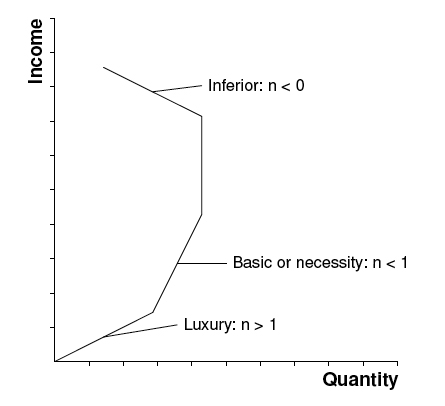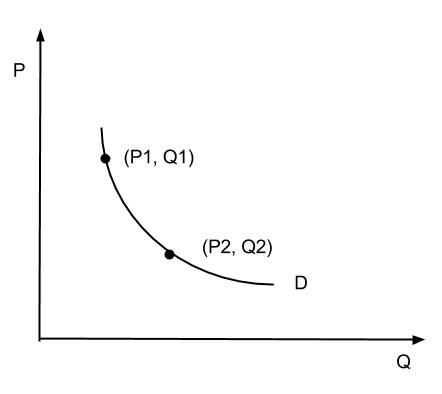## Cross-Price Elasticity of Demand

The cross-price elasticity of demand measures the change in demand for one good in response to a change in price of another good.

### Learning Objectives

Use the cross elasticity of demand to describe a good

### Key Takeaways

#### Key Points

• Complementary goods have a negative cross- price elasticity: as the price of one good increases, the demand for the second good decreases.
• Substitute goods have a positive cross-price elasticity: as the price of one good increases, the demand for the other good increases.
• Independent goods have a cross-price elasticity of zero: as the price of one good increases, the demand for the second good is unchanged.

#### Key Terms

• Complement: A good with a negative cross elasticity of demand, meaning the good’s demand is increased when the price of another good is decreased.
• substitute: A good with a positive cross elasticity of demand, meaning the good’s demand is increased when the price of another is increased.

The cross-price elasticity of demand shows the relationship between two goods or services. More specifically, it captures the responsiveness of the quantity demanded of one good to a change in price of another good. Cross-Price Elasticity of Demand (EA,B) is calculated with the following formula:

$E_{A,B} = \frac { \% Change\; in\; Quantity\; Demanded\; for\; Good\; A }{ \%\; Change\; in\; Price\; of\; Good\; B }$

The cross-price elasticity may be a positive or negative value, depending on whether the goods are complements or substitutes. If two products are complements, an increase in demand for one is accompanied by an increase in the quantity demanded of the other. For example, an increase in demand for cars will lead to an increase in demand for fuel. If the price of the complement falls, the quantity demanded of the other good will increase. The value of the cross-price elasticity for complementary goods will thus be negative.Complements: Two goods that complement each other have a negative cross elasticity of demand: as the price of good Y rises, the demand for good X falls.

A positive cross-price elasticity value indicates that the two goods are substitutes. For substitute goods, as the price of one good rises, the demand for the substitute good increases. For example, if the price of coffee increases, consumers may purchase less coffee and more tea. Conversely, the demand for a substitute good falls when the price of another good is decreased. In the case of perfect substitutes, the cross elasticity of demand will be equal to positive infinity.Substitutes: Two goods that are substitutes have a positive cross elasticity of demand: as the price of good Y rises, the demand for good X rises.

Two goods may also be independent of each other. In this instance, if the price of one good changes, demand for the other good will stay constant. For independent goods, the cross-price elasticity of demand is zero: the change in the price of one good with not be reflected in the quantity demanded of the other.Independent: Two goods that are independent have a zero cross elasticity of demand: as the price of good Y rises, the demand for good X stays constant.

## Income Elasticity of Demand

The income elasticity of demand measures the responsiveness of the demand for a good or service to a change in income.

### Learning Objectives

Analyze the characteristics of the income elasticity of demand.

### Key Takeaways

#### Key Points

• The income elasticity of demand is the ratio of the percentage change in demand to the percentage change in income.
• Normal goods have a positive income elasticity of demand (as income increases, the quantity demanded increases).
• Inferior goods have a negative income elasticity of demand (as income increases, the quantity demanded decreases).

#### Key Terms

• Necessary Good: A type of normal good. An increase in income leads to a smaller than proportional increase in the quantity demanded.
• Superior Good: A type of normal good. Demand increases more than proportionally as income rises.

The income elasticity of demand (YED) measures the responsiveness of demand for a good to a change in the income of the people demanding that good, ceteris paribus. It is calculated as the ratio of the percentage change in demand to the percentage change in income:

$YED=\quad \frac { \%\quad change\quad in\quad quantity\quad demanded }{ \%\quad change\quad in\quad real\quad income }$

If an increase in income leads to an increase in demand, the income elasticity of that good or service is positive. A positive income elasticity is associated with normal goods. In contrast, if a rise in income leads to a decrease in demand, the good or service has a negative income elasticity of demand. A negative income elasticity is associated with inferior goods.

In all, there are five types of income elasticity of demand:Income Elasticity of Demand: Income elasticity of demand measures the percentage change in quantity demanded as income changes.

• High income elasticity of demand (YED>1): An increase in income is accompanied by a proportionally larger increase in quantity demanded. This is typical of a luxury or superior good.
• Unitary income elasticity of demand (YED=1): An increase in income is accompanied by a proportional increase in quantity demanded.
• Low income elasticity of demand (YED<1): An increase in income is accompanied by less than a proportional increase in quantity demanded. This is characteristic of a necessary good.
• Zero income elasticity of demand (YED=0): A change in income has no effect on the quantity bought. These are called sticky goods.
• Negative income elasticity of demand (YED<0): An increase in income is accompanied by a decrease in the quantity demanded. This is an inferior good (all other goods are normal goods). The consumer may be selecting more luxurious substitutes as a result of the increase in income.

## Calculating Elasticities

The basic elasticity formula has shortcomings which can be minimized by using the midpoint method or calculating the point elasticity.

### Learning Objectives

Calculate price elasticity of demand with the midpoint method

### Key Takeaways

#### Key Points

• When changes in price and quantity are big, the arc elasticity or point elasticity formulas provide a more accurate elasticity coefficient than the basic elasticity formula.
• The arc elasticity captures the responsiveness of one variable to another between two given points.
• The midpoint method can be used if just two points on the demand curve are known. You do not need to know the function relating price and quantity demanded to use this method.
• The point elasticity captures the change in quantity demanded to a tiny change in price. To calculate the point elasticity, you must have a function for the relationship between price and quantity.

#### Key Terms

• Point elasticity: The measure of the change in quantity demanded to a very small change in price.
• Arc elasticity: The elasticity of one variable with respect to another between two given points.

The basic formula for the price elasticity of demand (percentage change in quantity demanded divided by the percentage change in price) yields an accurate result when the changes in quantity and price are small. As the difference between the two prices or quantities increases, however, the accuracy of the formula decreases. This happens because the price elasticity of demand often varies at different points along the demand curve and because the percentage change is not symmetric. Instead, the percentage change between any two values depends on which is chosen as the starting value. For example, when the quantity demanded increases from 10 units to 15 units, the percentage change is 50%. If the quantity demanded decreases from 15 units to 10 units, the percentage change is -33.3%. Two alternative elasticity measures can be used to avoid or minimize the shortcomings of the basic elasticity formula.

The midpoint method calculates the arc elasticity, which is the elasticity of one variable with respect to another between two given points on the demand curve. This measure requires just two points for quantity demanded and price to be known; it does not require a function for the relationship. The midpoint method uses the midpoint rather than the initial point for calculating percentage change, so it is symmetric with respect to the two prices and quantities demanded. The arc elasticity is obtained using this formula:Arc Elasticity: To calculate the arc elasticity, you need to know two points on the demand curve. The calculation does not require a function for the relationship between price and quantity demanded.

$Price\quad Elasticity\quad of\quad Demand\quad =\quad \frac { ({ Q }_{ 2 }-{ Q }_{ 1 })\quad /\quad [({ Q }_{ 2 }+{ Q }_{ 1 })/2] }{ ({ P }_{ 2 }-{ P }_{ 1 })\quad /\quad [(P_{ 2 }+{ P }_{ 1 })/2] }$

Suppose that the price of hot dogs changes from $3 to$1, leading to a change in quantity demanded from 80 to 120. The formula provided above would yield an elasticity of 0.4/(-1) = -0.4. As elasticity is often expressed without the negative sign, it can be said that the demand for hot dogs has an elasticity of 0.4.

The point elasticity is the measure of the change in quantity demanded to a tiny change in price. It is the limit of the arc elasticity as the distance between the two points approaches zero, and hence is defined as a single point. In contrast to the midpoint method, calculating the point elasticity requires a defined function for the relationship between price and quantity demanded. The point elasticity can be calculated with the following formula:

$Point-Price\quad Elasticity\quad =\quad \frac { P }{ { Q }_{ d } } \times \frac { \Delta { Q }_{ d } }{ \Delta P }$

In the formula above, dQ/dP is the partial derivative of quantity with respect to price, and P and Q are price and quantity, respectively, at a given point on the demand curve.